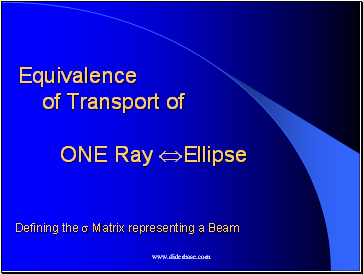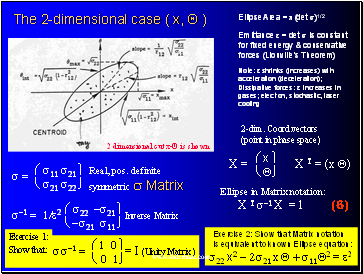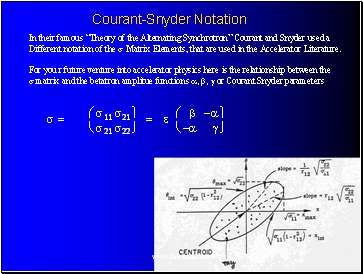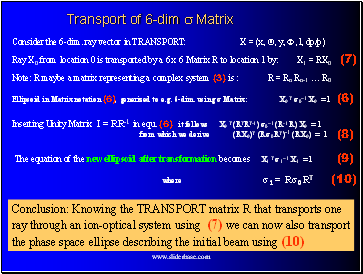# An Introduction to Ion-OpticsPage 4

#### WATCH ALL SLIDES

(Ellipse Area = p(det s)1/2

Emittance e is constant for

fixed energy & conservative

forces (Liouville’s Theorem)

Note: e shrinks (increases) with acceleration (deceleration); Dissipative forces: e increases in gases; electron, stochastic, laser cooling

Attention:

Space charge effects occur when the particle density is high, so that particles repel each other

Warning:

This is a mathematical abstraction of a beam:

2 dimensional cut x-Q is shown

e = Ö

s11s22 - (s12) 2

```````````

Emittance

(5)

Slide 17Equivalence of Transport of ONE Ray Û Ellipse

Defining the s Matrix representing a Beam

Slide 18## The 2-dimensional case ( x, Q )

Ellipse Area = p(det s)1/2

Emittance e = det s is constant

for fixed energy & conservative

forces (Liouville’s Theorem)

Note: e shrinks (increases) with acceleration (deceleration); Dissipative forces: e increases in gases; electron, stochastic, laser cooling

2 dimensional cut x-Q is shown

s =

æs11 s21 ü

ès21 s22 þ

Real, pos. definite

symmetric s Matrix

s-1 = 1/e2

æs22 -s21 ü

è-s21 s11 þ

Inverse Matrix

ss-1 =

æ1 0 ü

è 0 1þ

Exercise 1:

Show that:

= I (Unity Matrix)

2-dim. Coord.vectors

(point in phase space)

X =

X T = (x Q)

æx ü

èQþ

Ellipse in Matrix notation:

X T s-1 X = 1

Exercise 2: Show that Matrix notation

is equivalent to known Ellipse equation:

s22 x2 - 2s21 x Q + s11Q2 = e2

(6)

Slide 19## Courant-Snyder Notation

s =

æs11 s21 ü

ès21 s22 þ

In their famous “Theory of the Alternating Synchrotron” Courant and Snyder used a

Different notation of the s Matrix Elements, that are used in the Accelerator Literature.

For you r future venture into accelerator physics here is the relationship between the

s matrix and the betatron amplitue functions a, b, g or Courant Snyder parameters

æ b -a ü

è-a g þ

= e

Slide 20## Transport of 6-dim s Matrix

Consider the 6-dim. ray vector in TRANSPORT: X = (x, Q, y, F, l, dp/p)

Ray X0 from location 0 is transported by a 6 x 6 Matrix R to location 1 by: X1 = RX0

Note: R maybe a matrix representing a complex system (3) is : R = Rn Rn-1 … R0

Ellipsoid in Matrix notation (6), generized to e.g. 6-dim. using s Matrix: X0 T s0-1 X0 = 1

Go to page:
1  2  3  4  5  6